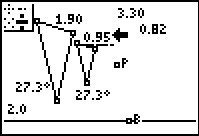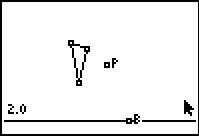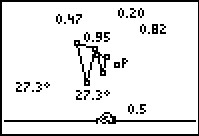••• ##### Device
• TI-83 Plus Family
• TI-84 Plus
• TI-84 Plus Silver Edition
•TI-84 Plus C Silver Edition
•TI-84 Plus CE
• ##### Software

TI Connect™
TI Connect™ CE

• ##### Report an Issue

Geometry: Scale Factor
by Texas Instruments#### Overview

Students dilate polygons and find the perimeter and area of both the pre-image and image.

#### Key Steps

•In Problem 1, students use the file SLIDER to investigate scale factor and dilations. They construct a small scalene triangle using the Triangle tool. They use the Point tool and create a point in the middle of the screen.

•Students will use the Dilation command to dilate a figure at a point using a scale factor of 2. They can then find the ratios of the perimeter and the area of the image to that of the pre-image.

Students set up expressions for the ratios. They can drag the vertices of the pre-image and observe changes in the image. They see that the ratio of the perimeters is equal to the scale factor, and the ratio of the areas is equal to the square of the scale factor.

•In Problem 2, students explore another type of dilation called a reduction. Students drag the point so the scale factor can be change to 0.5. Students will see that the ratios of the perimeter and the area of the image to that of the pre-image.